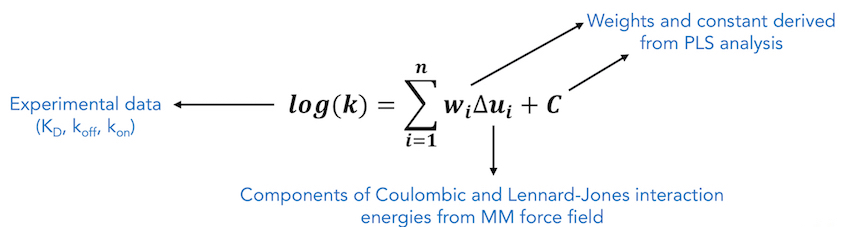### Introduction

COMBINE analysis uses partial least squares regression to derive a system-specific model for binding free energy or a related experimental observable based on weighting of force field energy terms describing contributions to the protein - ligand interaction energy.

COMBINE approach is used to derive a target-specific scoring function to compute binding free energy or a related property by exploiting the information contained in the 3D structures of receptor-ligand complexes. Energy-minimized structures of protein-ligand complexes are used to compute protein - ligand interaction energies using a molecular mechanics force field. These energies are then partitioned and subjected to regression based methods such as Partial Least Squares (PLS) regression, to derive a statistical model which relates the experimental observable to weighted selected components of the protein - ligand interaction energy. The interaction energy components are typically Lennard-Jones and Coulombic terms from a standard force field, decomposed on a per residue basis.References:

1. Ortiz a R, Pisabarro MT, Gago F, Wade RC. Prediction of drug binding affinities by comparative binding energy analysis. J Med Chem. 1995;38: 2681–2691.
2. Murcia M, Morreale A, Ortiz AR. Comparative binding energy analysis considering multiple receptors: A step toward 3D-QSAR models for multiple targets. J Med Chem. 2006;49: 6241–6253.

### Example Cases

For examples of previously performed studies in which Comparative Binding Energy (COMBINE) Analysis was the primary method used, see the following example cases:

### Tutorials

The folowing tutorial describes the use of Comparative Binding Energy (COMBINE) Analysis: